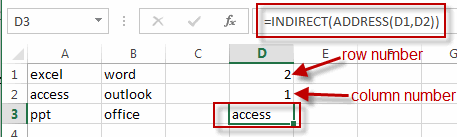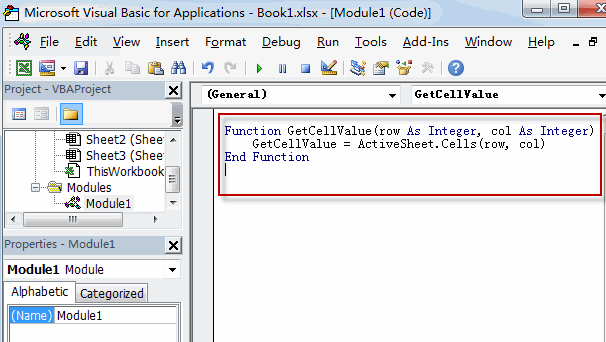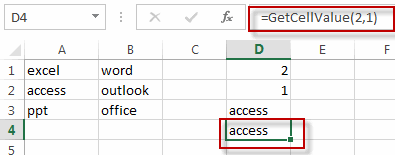# Get Cell Value Based on Row and Column Numbers

This post will guide you how to retrieve values using references based on Row and Column numbers in excel. How to get the cell value based on row and column numbers with an excel formula or user defined function in excel.

## Get Cell Value Based on Row and Column Numbers

If you want to retrieve values using cell references based on row and column numbers, you can create a formula based on the INDIRECT function and the ADDRESS function.

Assuming that there is a row number in cell D1 and there is a column number in cell D2, if you want to retrieve the cell value based on the specified row number and column number, just type the following formula in cell D3:

`=INDIRECT(ADDRESS(D1,D2))`You can also write a User Defined Function to get content of a cell given the row and column numbers. Just do the following steps:

#1 open your excel workbook and then click on “Visual Basic” command under DEVELOPER Tab, or just press “ALT+F11” shortcut.#2 then the “Visual Basic Editor” window will appear.

#3 click “Insert” ->”Module” to create a new module#4 paste the below VBA code into the code window. Then clicking “Save” button.```Function GetCellValue(row As Integer, col As Integer)
GetCellValue = ActiveSheet.Cells(row, col)
End Function```

#5 back to the current worksheet, try to enter the below formula in Cell D4.

`=GetCellValue(2,1)`